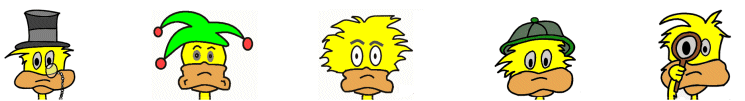# Kids Math

## Finding the Volume and Surface Area of a Cylinder

What is a cylinder?

There are different kinds of cylinders. On this page we will be discussing the most simple form where the cylinder looks like a tube or a soup can with two circles at each end that are the same size and parallel.Terms of a Cylinder

In order to calculate the surface area and volume of a cylinder we first need to understand a few terms:

Radius - The radius is the distance from the center to the edge of the circles at each end.

Pi - Pi is a special number used with circles. We will use an abbreviated version where Pi = 3.14. We also use the symbol π to refer to the number pi in formulas.

Height - The height or length of the cylinder.

Surface Area of a Cylinder

The surface area of a cylinder is the surface area of both circles at each end plus the surface area of the outside of the tube. There is a special formula used to figure this out.

Surface area = 2πr2 + 2πrh

h = height
π = 3.14

This is the same as saying (2 x 3.14 x radius x radius) + (2 x 3.14 x radius x height)

Example:

What is the surface area of a cylinder with radius 3 cm and height 5 cm?

Surface area = 2πr2 + 2πrh
= (2x3.14x3x3) + (2x3.14x3x5)
= 56.52 + 94.2
= 150.72 cm2

Volume of a Cylinder

There is special formula for finding the volume of a cylinder. The volume is how much space takes up the inside of a cylinder. The answer to a volume question is always in cubic units.

Volume = πr2h

This is the same as 3.14 x radius x radius x height

Example:

Find the volume of a cylinder with radius 3 cm and height 5 cm?

Volume = πr2h
= 3.14 x 3 x 3 x 5
= 141.3 cm 3

Things to Remember
• Surface area of a cylinder = 2πr2 + 2πrh
• Volume of a cylinder = πr2h
• You need to know the radius and height to figure both the volume and surface area of a cylinder.
• Answers for volume problems should always be in cubic units.
• Answers for surface area problems should always be in square units.

More Geometry Subjects

Circle
Polygons
Triangles
Pythagorean Theorem
Perimeter
Slope
Surface Area
Volume of a Box or Cube
Volume and Surface Area of a Sphere
Volume and Surface Area of a Cylinder
Volume and Surface Area of a Cone
Angles glossary
Figures and Shapes glossary

Back to Kids Math

Back to Kids Study